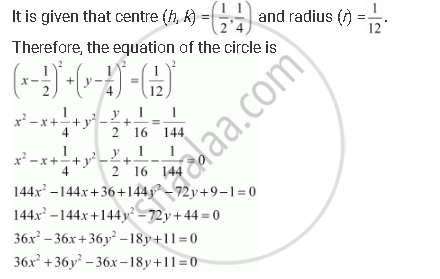Share

# Find the Equation of the Circle with (1/2, 1/4)And Radius 1/12 - Mathematics

#### Question

Find the equation of the circle with (1/2, 1/4)and radius 1/12

#### Solution

The equation of a circle with centre (hk) and radius is given as

(x­ – h)2 + (y ­– k)2 = r2Is there an error in this question or solution?